×#### Thank you for registering.

One of our academic counsellors will contact you within 1 working day.

Click to Chat

1800-1023-196

+91-120-4616500

CART 0

• 0

MY CART (5)

Use Coupon: CART20 and get 20% off on all online Study Material

ITEM
DETAILS
MRP
DISCOUNT
FINAL PRICE
Total Price: Rs.

There are no items in this cart.
Continue ShoppingDegree of Dissociation

Table of Content

Degree of Dissociation

Dependence of Degree of Dissociation from Density Measurements

Thermodynamics of Chemical Equilibrium

Related Resources

Degree of Dissociation ()The degree of dissociation of a substance is defined as the fraction of its molecules dissociating at a given time.

Let us consider the reaction,

2NH3 (g)  N2 (g) + 3H2 (g)

Let the initial moles of NH3(g) be ‘a’. Let  x moles of NH3 dissociate at equilibrium.Degree of dissociation (a) of NH3 is defined as the number of moles of NH3 dissociated per mole of NH3.

if x moles dissociate from ‘a’ moles of NH3, then, the degree of dissociation of NH3 would be x/a.

We can also look at the reaction in the following manner.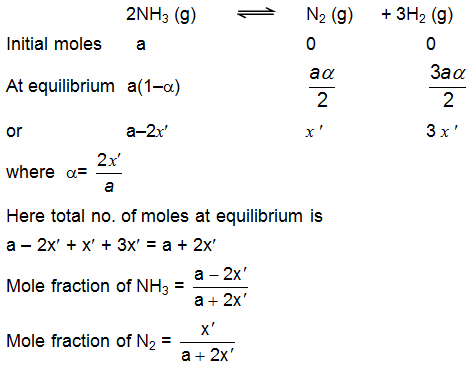In this way you should calculate the basic equation. So my advice to you is that, while solving problem follow the method given below:

Write the balanced chemical reaction (mostly it will be given)

Under each component write the initial no. of moles.

Do the same for equilibrium condition.

Then derive the expression.

Dependence of Degree of Dissociation from Density Measurements

?Refer to the following video for Vapour density and degree of dissociation

The following is the method of calculating the degree of dissociation of a gas using vapour densities. This method is valid only for reactions whose KP exist, i.e., reactions having at least one gas and having no solution.

Since

pV = nRT

pV = nRT

Since

P = NRT / V

For a reaction at equilibrium V is a constant and ρ is a constant.   vapour Density α 1/n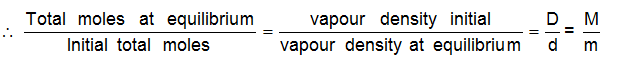(molecular weight = 2 × V.D)

Here  M = molecular weight initial

m = molecular weight at equilibrium

Let us take a reaction

PCl5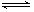PCl3    +   Cl2

Initial moles      C                      0                0

At eqb.            C(1-α)              Cα              Cα

∴  = C(1+α) / C = D/d;     1 + α = D/d = M/m

Knowing D and d, α can be calculated and so for M and m.

Thermodynamics of Chemical Equilibrium

Let ΔG0 be the difference in free energy of the reaction when all the reactants and products are in the standard state and Kc or, Kp be the thermodynamic equilibrium constant of the reaction. Both related to each other at temperature T by the following relation.

ΔG0 = – 2.303 RT logKc and ΔG0 = – 2.303 RT log Kp(in case of ideal gases)

Now, we know that thermodynamically,

ΔG0 = ΔH0 – TDS0

here ΔH0 is standard enthalpy of reaction, and ΔS0 is standard entropy change

(i) When ΔG0 = 0, then, Kc = 1

(ii) When, ΔG0 > 0, i.e. +ve, then Kc < 1, in this case reverse reaction is feasible showing thereby a less concentration of products at equilibrium rate.

(iii) When ΔG0 < 0, i.e. -ve, then, Kc > 1; In this case, forward reaction is feasible showing thereby a large concentrations of product at equilibrium state.

At equilibrium,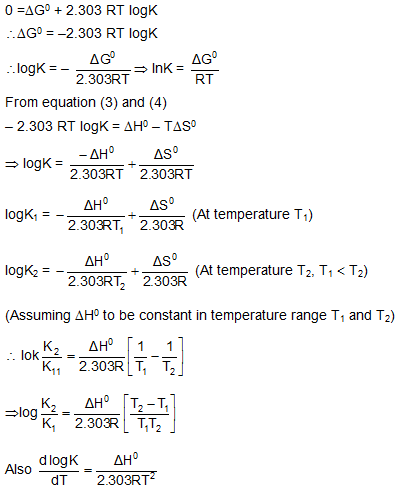Example 1.  When PCl5 is heated it dissociates into PCl3 and Cl2. The density of the gas mixture at 200oC and at 250oC is 70.2 and 57.9 respectively. Find the degree of dissociation at 200oC and 250oC.

Solution: PCl5(g) →  PCl3(g) + Cl2(g)

We are given the vapour densities at equilibrium at 200oC and 250oC.

The initial vapour density will be the same at both the temperatures as

it would be  MPCl5 / 2.

∴ Initial vapour density = (31 + 5 × 35.5) / 2 = 104.25

Vapour density at equilibrium at 200oC = 70.2

∴ Total moles at equilibrium / Total moles initial  = 1 + α = Vapour density initial / Vapour density at equilibrium     = 104.25 / 70.2 = 1.485

∴  a = 0.485

At 250oC,       1 + α = 104.25 / 57.9 = 1.8

∴  a = 0.8

Sample problem.

The degree of dissociation of PCl5 is 60%, then find out the observed molar mass of the mixture.

Solution:     PCl5(g)PCl3(g) + Cl2(g)

Initially moles           1                  0         0

At eqm.                 1 – α               α         α

Where α = degree of dissociation = 0.6

Total moles at equilibrium = 1 + a = observed mole

1 + a = 206.5 / molecular weight observed

molecular weight observed = 206.5 / 1 + a = 206.5 / 1.6 = 129.06

Example 2. A system is in equilibrium as  PCl5 + Heat —> PCl3 + Cl2

Why does the temperature of the system decreases, when PCl3 are being removed from the equilibrium mixture at constant volume?

Solution:  When PCl3 are being removed from the system, the reaction moved to the right. This consumed heat and therefore, temperature is decreased.

Example. NO and Br2 at initial partial pressures of 98.4 and 41.3 torr, respectively, were allowed to react at 300K. At equilibrium the total pressure was 110.5 torr. Calculate the value of the equilibrium constant and the standard free energy change at 300K for the reaction 2NO(g) + Br2(g) 2NOBr(g).

Solution:

2NO(g) + Br2(g) →  2NOBr(g)

Initial pressure         98.4          41.3                 0

At eqlbm.                98.4-x       41.3 - x/2          x

The total pressure at equilibrium is 110.5 torr.

98.4-x + 41.3- x/2 + x = 110.5

x = 58.4 torr

Now, 1 atm = 760.4 torr,

x = 7.68 × 10-2 atm.

pNOBr = 7.68 × 10-2 atm ;     pNO = 98.4-x = 40 torr = 5.26 × 10-2 atm.

pBr2 = 41.3 – x/2 = 12.1 torr = 1.59 × 10-2 atm.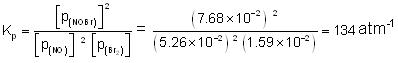ΔGo = -2.303 RT log K = -2.303 (1.99) (300) (log 134)

= -2.92 k cal = -12.2 kJ.

[If R is used as 1.99 cal/mol K, then ΔGo will be in cal. If R is used as 8.314 J/mol K, then ΔGo will be in Joules. But KP must always be in (atm)Δn.]

Related Resources

JEE  Chemistry Syllabus

Chemistry Reference Books

To read more, Buy study materials of Chemical Equilibrium comprising study notes, revision notes, video lectures, previous year solved questions etc. Also browse for more study materials on Chemistry here.### Course Features

• 731 Video Lectures
• Revision Notes
• Previous Year Papers
• Mind Map
• Study Planner
• NCERT Solutions
• Discussion Forum
• Test paper with Video Solution